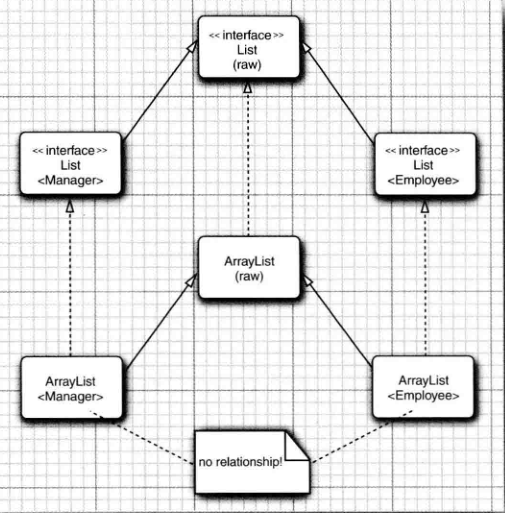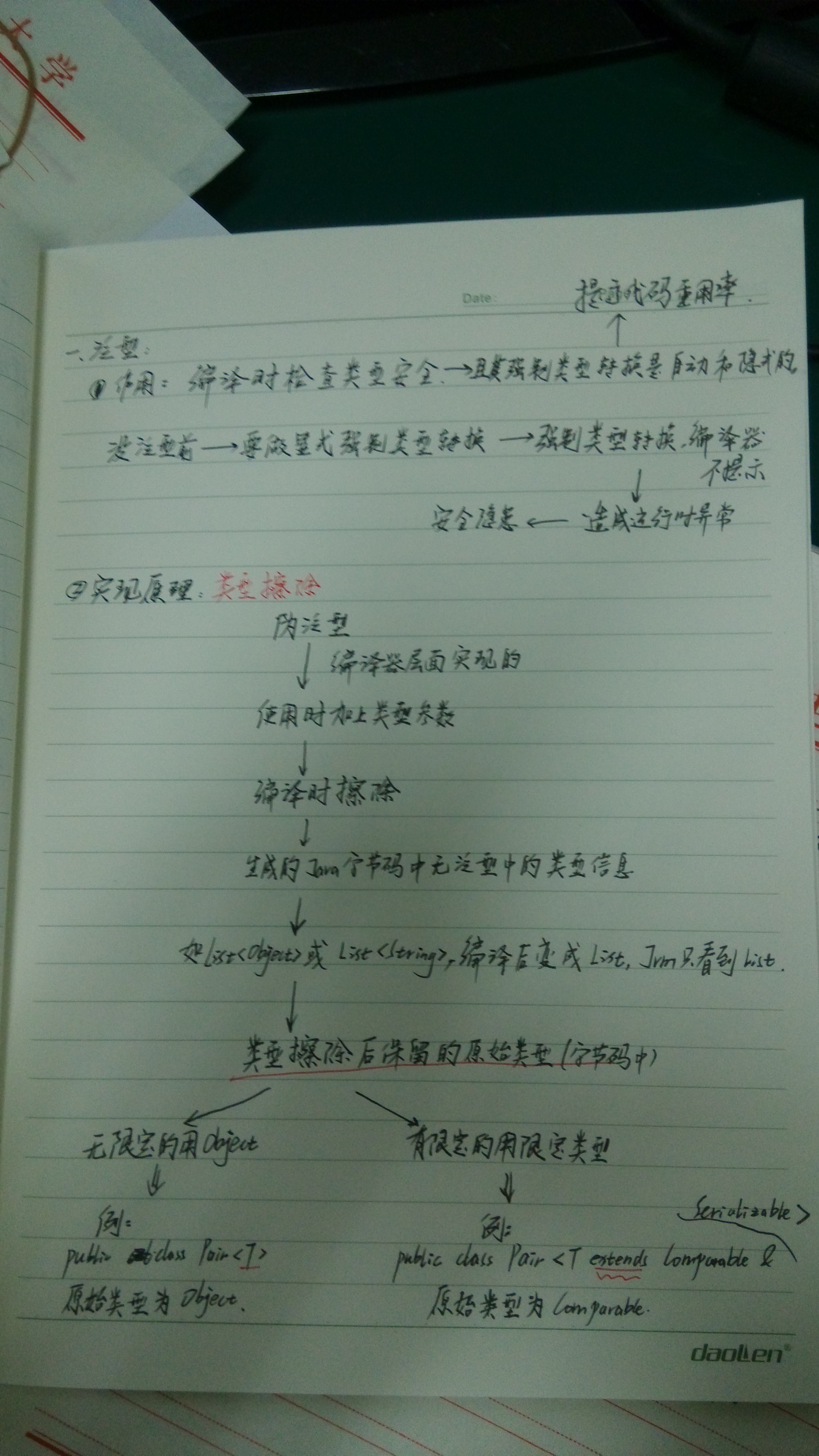# 泛型三种形式

``````public <T extends Comparable & Serializable> void method(T[] strs) {
T a = strs;
T b = strs;
a.compareTo(b);
}``````

## 泛型继承规则``````public interface A<T> {
void a(T a);
}
public class B<T> implements A<T> {
@Override
public void a(T a) {
System.out.println(this);
}
}
public class Pair<T> {
void  print(Pair<T> pair) {
System.out.println(pair);
}
}
public class Test {

public static void main(String[] args) {
Pair<A> pairA = new Pair<>();
Pair<B> pairB = new Pair<>();
pairA.print(pairB); // 这里编译会出错。
}
}``````

## 通配符类型

``````public class Pair<T> {
void  print(Pair<? extends T> pair) {
System.out.println(pair);
}
}
public class Test {
public static void main(String[] args) {
Pair<A> pairA = new Pair<>();
Pair<B> pairB = new Pair<>();
pairA.print(pairB); // 这里编译会通过。
}
}``````

## 无限定通配符

还可以使用无限定的通配符 ， 例如， Pair < ? > 。 初看起来 ， 这好像与原始的 Pair 类型一样 。实际上 ，有很大的不同。 类型Pair < ? > 有以下方法 ：

``````? getFirst ( )
void set First (?)``````

getFirst 的返回值只能赋给一个 Object 。 setFirst 方法不能被调用， 甚至不能用Object 调用 。 Pair < ? > 和 Pair 本质的不同在于 ： 可以用任意 Object 对象调用原始 Pair 类的 setObject方法。

可以调用 setFirst ( null ) 。为什么要使用这样脆弱的类型 ？ 它对于许多简单的操作非常有用。 例如 ， 下面这个方法将用来测试一个 pair 是否包含一个 null 引用， 它不需要实际的类型 。

``````public static boolean hasNulls (Pair<?> p) {
return p.getFirst() = null || p.getSecond() = null ;
}``````

``public static < T > boolean hasNulls ( Pair < T > p )``

``````public static void swap (Pair <?> p) {
? t = p.getFirst() ; // Error, 编译错误。
p.setFirst(p.getSecond());
p.setSecond(t);
}``````

``````public static <T> void swapHelper (Pair <T> p){
T t = p.getFirst();
p.setFirst(p.getSecond());
p.setSecond(t) ;
}``````

## 泛型实现原理：类型擦除

``Assert.assertTrue(new ArrayList<String>().getClass() == new ArrayList<Integer>().getClass());``

``````    public static void main(String[] args) {
new Test().method(new Integer[]{1,2});
}

public <T extends Comparable & Serializable> void method(T[] strs) {
T a = strs;
T b = strs;
System.out.println(a.compareTo(b));
}``````
``````  public <T extends java.lang.Comparable & java.io.Serializable> void method(T[]
);
descriptor: ([Ljava/lang/Comparable;)V
flags: ACC_PUBLIC
Code:
stack=3, locals=5, args_size=2
1: iconst_0
3: astore_2
5: iconst_1
7: astore_3
9: checkcast     #7                  // class java/io/Serializable
12: astore        4
14: getstatic     #8                  // Field java/lang/System.out:Ljav
a/io/PrintStream;
20: invokeinterface #9,  2            // InterfaceMethod java/lang/Compa
rable.compareTo:(Ljava/lang/Object;)I
25: invokevirtual #10                 // Method java/io/PrintStream.prin
tln:(I)V
28: return
LineNumberTable:
line 21: 0
line 22: 4
line 23: 8
line 24: 14
line 25: 28
LocalVariableTable:
Start  Length  Slot  Name   Signature
0      29     0  this   Lcom/leetcode/tal/template/Test;
0      29     1  strs   [Ljava/lang/Comparable;
4      25     2     a   Ljava/lang/Comparable;
8      21     3     b   Ljava/lang/Comparable;
14      15     4     s   Ljava/io/Serializable;
LocalVariableTypeTable:
Start  Length  Slot  Name   Signature
0      29     1  strs   [TT;
4      25     2     a   TT;
8      21     3     b   TT;
Signature: #36                          // <T::Ljava/lang/Comparable;:Ljava/
io/Serializable;>([TT;)V
}``````### 特殊的擦除：桥方法

``````class Datelnterval extends Pair <LocalDate> {
public void setSecond() {
if ( second.compareTo(getFirst()) >= 0 )
super.setSecond(second);
}
}``````

``````class Datelnterval extends Pair // after erasure
{
public void setSecond ( LocalDate second ) { . . . }
}``````

``````Datelnterval interval = new Datelnterval ( . . . ) ;
Pair <LocalDate> pair = interval; / / OK assignment to superclass，raw type也是它

public void setSecond ( Object second ) { setSecond ( ( Date ) second ) ;

``````class Datelnterval extends Pair <LocalDate>{
public Local Date getSecond() { return (Date)super.getSecond().clone();
}``````

• Local Date getSecond ( ) / / defined in Datelnterval
• Object getSecond 0 // overrides the method defined in Pair to call the first method

## 泛型的限制

• 不能用基本类型实例化类型参数。如Class<int>, Class<double>是不允许的，只能Class<Integer>, Class<Double>
• 运行时类型查询只适用于原始类型。如if ( a instanceof Class<String> ) 是不允许的。
• 不能创建参数化类型的数组。如Pair<String>[] table = new Pair<String> ; 也是不允许的。

Object [] objarray = table ;

obj array [ 0 ] = " Hello " ; // Error component type is Pair

Pair <String>[] table = (Pair<String>[]) new Pair<?>

如果需要收集参数化类型对象 ， 只有一种安全而有效的方法 ： 使用 ArrayList : ArrayList <Pair<String>>

• Varargs 警告

``````public static <T> void addAll(Collections coll , T . . . ts ){
}``````

``````Col 1 ection <Pair <String>> table = . . . ;
Pair<String> pairl = . . . ;
Pair<String> pair2 = . .

``````@SafeVarargs
public static < T > void addAll ( Collection < T > coll , T ... ts )``````

• 不能实例化类型变置。如public Pair ( ) { first = new T () ; second = new T ( ) ; } 是不允许的。

在 Java SE 8 之后 ，最好的解决办法是让调用者提供一个构造器表达式。 例如 ：
Pair < String > p = Pair.makePair(String : : new) ;
makePair 方法接收一个 Supplier < T >， 这是一个函数式接口 ， 表示一个无参数而且返回类型为 T 的函数 ：

``````public static <T> Pair <T> makePair(Supplier <T> constr){
return new Pair <>(constr.get())；
}``````

``````public static <T> Pair <T> makePair (Class <T> cl) {
try { return new Pair<>(cl.newInstance())； }
catch ( Exception ex ) { return null; }
}``````
• 不能构造泛型数组
• 泛型类的静态上下文中类型变量无效
• 不能抛出或捕获泛型类的实例
• 可以消除对受查异常的检查

## 总之

Java 泛型转换的事实 ：
• 虚拟机中没有泛型 ， 只有普通的类和方法 。
• 所有的类型参数都用它们的限定类型替换 。
• 桥方法被合成来保持多态 。
• 为保持类型安全性 ， 必要时插人强制类型转换 。